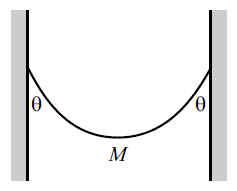# JEE Mains Mechanics (Equilibrium) 1A chain of mass 2 Kg hangs between two walls, with its ends at the same height. The chain makes an angle of $\theta=60^\circ$ with each wall, as shown in figure. Find the tension in the chain at the lowest point (mid-point). $(g=9.8\ SI units)$

×